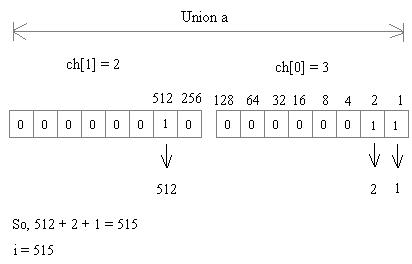# C Programming - Declarations and Initializations

Exercise : Declarations and Initializations - Find Output of Program
6.
What will be the output of the program?
``````#include<stdio.h>
int X=40;
int main()
{
int X=20;
printf("%d\n", X);
return 0;
}
``````
20
40
Error
No Output
Explanation:
Whenever there is conflict between a local variable and global variable, the local variable gets priority.

7.
What is the output of the program
``````#include<stdio.h>
int main()
{
int x = 10, y = 20, z = 5, i;
i = x < y < z;
printf("%d\n", i);
return 0;
}
``````
0
1
Error
None of these
Explanation:
Since x < y turns to be TRUE it is replaced by 1. Then 1 < z is compared and to be TRUE. The 1 is assigned to i.

8.
What is the output of the program
``````#include<stdio.h>
int main()
{
extern int fun(float);
int a;
a = fun(3.14);
printf("%d\n", a);
return 0;
}
int fun(int aa)
{
return (int)++aa;
}
``````
3
3.14
4
Compile Error
Explanation:
2 Errors
1. Type mismatch in redeclaration of fun
2. Type mismatch in parameter aa

9.
What is the output of the program
``````#include<stdio.h>
int main()
{
int a = {2, 3};
printf("%d, %d, %d\n", a, a, a);
return 0;
}
``````
Garbage Values
2, 3, 3
3, 2, 2
0, 0, 0
Explanation:
When an automatic array is partially initialized, the remaining elements are initialized to 0.

10.
What is the output of the program?
``````#include<stdio.h>
int main()
{
union a
{
int i;
char ch;
};
union a u;
u.ch = 3;
u.ch = 2;
printf("%d, %d, %d\n", u.ch, u.ch, u.i);
return 0;
}
``````
3, 2, 515
515, 2, 3
3, 2, 5
None of these